# Core Java. Lecture #5

## Regular expressions

``````String input = "11:59 am";
Pattern pattern =
Pattern.compile("([1-9]|1[0-2])\\s*:\\s*([0-5][0-9])\\s*([ap]m)");
Matcher matcher = pattern.matcher(input);

//matches() requires full match for the whole string,
//find() -- finds the first match
if (matcher.matches()) {
String hours = matcher.group(1); //11
String minutes = matcher.group(2); //59
String period = matcher.group(3); //am
}``````

## Find/replace in a loop

An example from StackOverflow: we want "1xxxx23" as an output:

``````Map<String, String> replacements = new HashMap<String, String>();

replacements.put("\${env1}", "1");
replacements.put("\${env2}", "2");
replacements.put("\${env3}", "3");

String line ="\${env1}xxxx\${env2}\${env3}";``````

## Find/replace in a loop

``````//Before Java9 StringBuffer was used
StringBuilder sb = new StringBuilder();

Pattern p = Pattern.compile("(\\\$\\{[^}]+\\})");
Matcher m = p.matcher(line);
while (m.find()) {
String repString = replacements.get(m.group(1));
if (repString != null)
m.appendReplacement(sb, repString);
}
m.appendTail(sb);

System.out.println(sb.toString()); //1xxxx23``````

## Regular expressions

• Can validate string format

• Tolerable to optional separators, 'garbage' etc.

• Groups are powerful device for data extraction

• The price we pay

• You have to learn (there are thick books on regular expressions)

• You have to learn to use them correctly (you can easily shatter the performance)

• They look horribly and perplexing

## State machines

How can we parse the following string?

``````  Name,Goods and Services
"LLC ""Sea Shore Shop""",sea shells``````

## Definite State Machine!## Implementation

``````enum State {START, QUOTED_BODY, QUOTE_READ, UNQUOTED_BODY}
List<String> parse(String line) {
State state = State.START;
List<String> result = new ArrayList<>();
StringBuilder fieldValue = new StringBuilder();
for (char c : line.toCharArray()) {
switch (state) {
case START: . . .
case QUOTED_BODY: . . .
case UNQUOTED_BODY: . . .
}
}
return result;
}``````

## Started to read the field``````case START:
fieldValue = new StringBuilder();
if (c == '"')
state = State.QUOTED_BODY;
else {
fieldValue.append(c);
state = State.UNQUOTED_BODY;
}
break;``````

## Quoted value``````case QUOTED_BODY:
if (c == '"')
else {
fieldValue.append(c);
state = State.QUOTED_BODY;
}
break;``````

## Came across a quote mark``````case QUOTE_READ:
if (c == '"') {
fieldValue.append(c);
state = State.QUOTED_BODY;
} else if (c == ',') {
state = State.START;
} else { //invalid input format
throw new IllegalStateException();
} break;``````

## Unquoted field``````case UNQUOTED_BODY:
if (c == ',') {
state = State.START;
} else {
fieldValue.append(c);
}
break;``````

## Conclusion

• Mathematical fact: Each FSM can be converted into a regular expression, and vice versa.

• When processing regular and FSM strings, that’s our everything.

## Wrapper Types

Primitive types are incompatible with objects. So there are Wrapper Classes:

 `byte`, `short`, `int`, `long` `Byte`, `Short`, `Integer`, `Long` `char` `Character` `float`, `double` `Float`, `Double` `boolean` `Boolean`

## Wrapper-types are similar to strings

• Objects are immutatable. If a new one is needed, we make a new object, not modify the old one.

• Equality check — with `equals` only!

• Like strings in string pool, these objects are cached, so it they should be created with `valueOf` rather than `new`.

## Object pool (caching)

 ``````Integer five1 = Integer.valueOf(5); Integer five2 = Integer.valueOf(5); /* DO NOT DO THIS!!*/ Integer five3 = new Integer(5);``````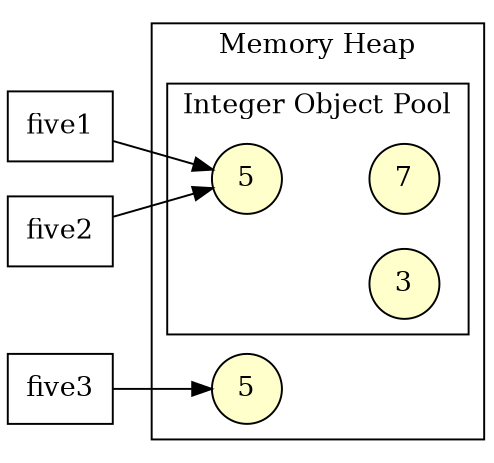## What is cached

• `Integer`, `Short`, `Long` — -128..127

• 'Byte' — completely (-128..127)

• `Character` — 0..127

• 'Boolean' — 'Boolean.TRUE' and 'Boolean.FALSE'

• 'Float', 'Double' — are not cached, although in the JavaDoc for `valueOf` the possible caching is mentioned.

• Truth of Life: You’ll use autoboxing more often than `valueOf`.

## Autoboxing & unboxing

``````ArrayList<Integer> list = new ArrayList<>();

//autoboxing :-)
//without autoboxing :-(

//unboxing :-)
int n = list.get(i);
//with no automatic unboxing :-(
int n = list.get(i).intValue();``````

## Autoboxing is not always that obvious

``````Integer n = 3;
//unbox - increment - box.
//not so effective!!
n++;

Integer a = 1000;
Integer b = 1000;
if (a == b) . . . //NO! THIS WILL FAIL!``````

## Convert a number from and to a string

Static methods:

• `Integer.parseInt(value, radix)`

• `Integer.toString(value, radix)`

• `Double.parseDouble(value)`

• `Double.toString(value)`

## `BigInteger` class

``````BigInteger i = new BigInteger("123456789012345678901234567890");
BigInteger j = new BigInteger("123456789012345678901234567892");

BigInteger difference = i.subtract(j);
BigInteger quotient = i.divide(j);
BigInteger product = i.multiply(j);``````

## `BigDecimal` class

``````public static BigDecimal calculateTotalAmount(
BigDecimal quantity,     BigDecimal unitPrice,
BigDecimal discountRate, BigDecimal taxRate) {
BigDecimal amount = quantity.multiply(unitPrice);
BigDecimal discount = amount.multiply(discountRate);
BigDecimal discountedAmount = amount.subtract(discount);
BigDecimal tax = discountedAmount.multiply(taxRate);

// round to 2 decimal places using HALF_EVEN
BigDecimal roundedTotal = total.setScale(2, RoundingMode.HALF_EVEN);
return roundedTotal;
}``````

## Input/OutputIBM2741 terminal, 1965 (source: Wikipedia)

## A complete zoo of I/O classes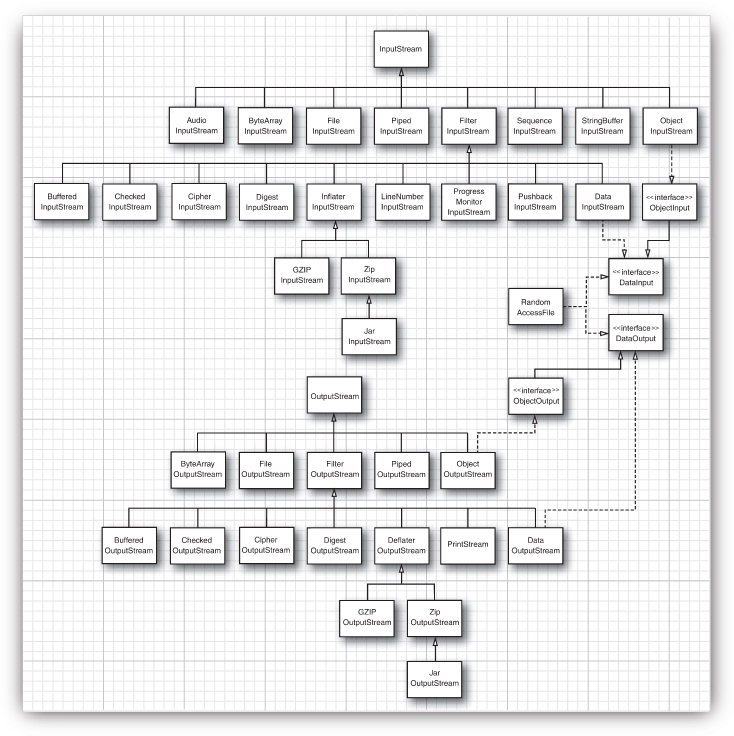Cay Horstmann. Core Java, vol. II

## Classes for text input/output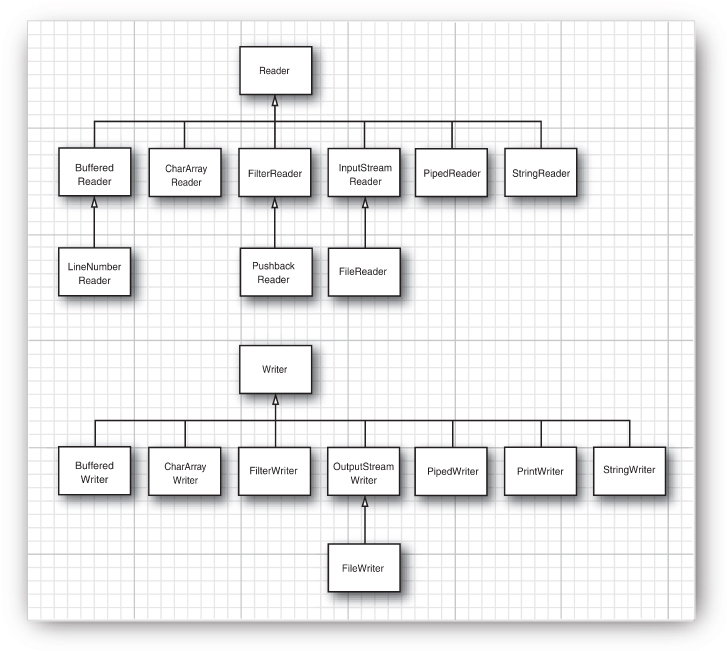Cay Horstmann. Core Java, vol. II

## Basic I/O streams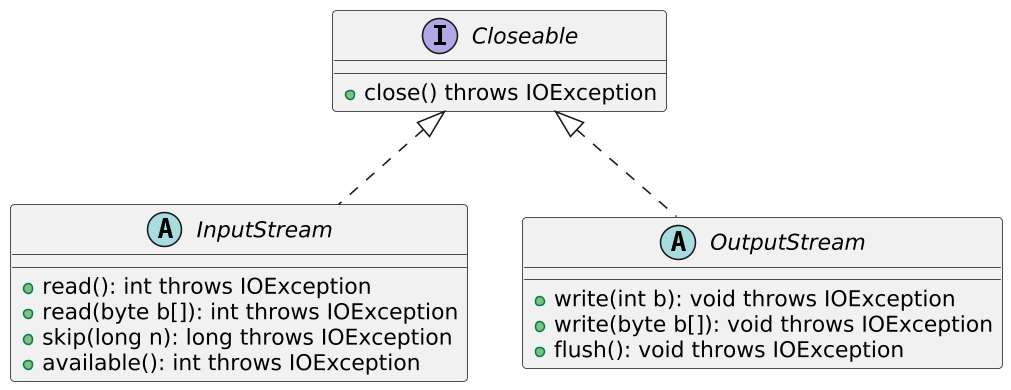## Combining streams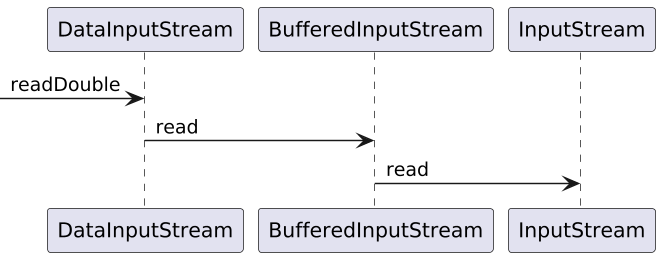``````Path path = Paths.get("path/to/file.dat");

try (DataInputStream dis =
new DataInputStream(
new BufferedInputStream(
Files.newInputStream(path)))) {
}``````

## Combining Streams: Reading a Zip File

``````Path path = Paths.get("path/to/file.zip");

try(ZipInputStream zis =  new ZipInputStream(
Files.newInputStream(path));
DataInputStream dis = new DataInputStream(zis)) {
. . .
}``````

The modern, simple and correct method:

``````Path path = Paths.get("path/to/file.txt");

Stream<String> lines = Files.lines(path, StandardCharsets.UTF_8);

//Reading of all the contents into memory
List<String> strings =

## Using `BufferedReader`

``````try (BufferedReader br =
String line;
. . .
}
}``````

## Save prepared data to text

``````Path path = Paths.get("path/to/file.txt");
List<String> lines = new List<String>();

//If everything is already in memory
Files.write(path, lines, StandardCharsets.UTF_8);``````

## Saving the text data incrementally

``````try (PrintWriter printWriter = new PrintWriter(
Files.newBufferedWriter(
path, StandardCharsets.UTF_8))) {
printWriter.println(...);
printWriter.printf(...);
}``````

## Working with the file system

• Obsolete `File` (can still be found, in particular, as an argument of the `FileInputStream` constructor).

• More modern `Path` (Java 7+)

## Working with the file system

``````//Path does not necessarily specify
//an existing file or directory!!

Path absolute = Paths.get("/home", "harry");
Path relative = Paths.get("myprog", "conf", "user.properties");

Path subPath = absolute.resolve("file.txt");

subPath.getParent(); //parent
subPath.getFileName(); //the last component of the path``````

## Working with the file system

``````//all components except the last component must exist
Files.createDirectory(path);
creates all directories in path
Files.createDirectories(path);
creates an empty file
Files.createFile(path);

//creates a temporary file in an OS-specific location
Files.createTempFile(prefix, suffix);``````

## File verification

``````//it makes sense to check before you open a stream for reading
Files.exists(path)

Files.size(path)``````

## Getting the list of files and depth-first directory traversal

``````//the contents of the folder (without going to subfolders)
Stream<Path> list = Files.list(path);

//traverse the folder tree
Stream<Path> Files.walk(Path start,
int maxDepth, FileVisitOption... options)``````

## Copy, move and delete files

``````Files.copy(fromPath, toPath)

Files.move(fromPath, toPath)

Files.delete(path)``````

## Clocks and Datetime API

How many seconds are there in a day?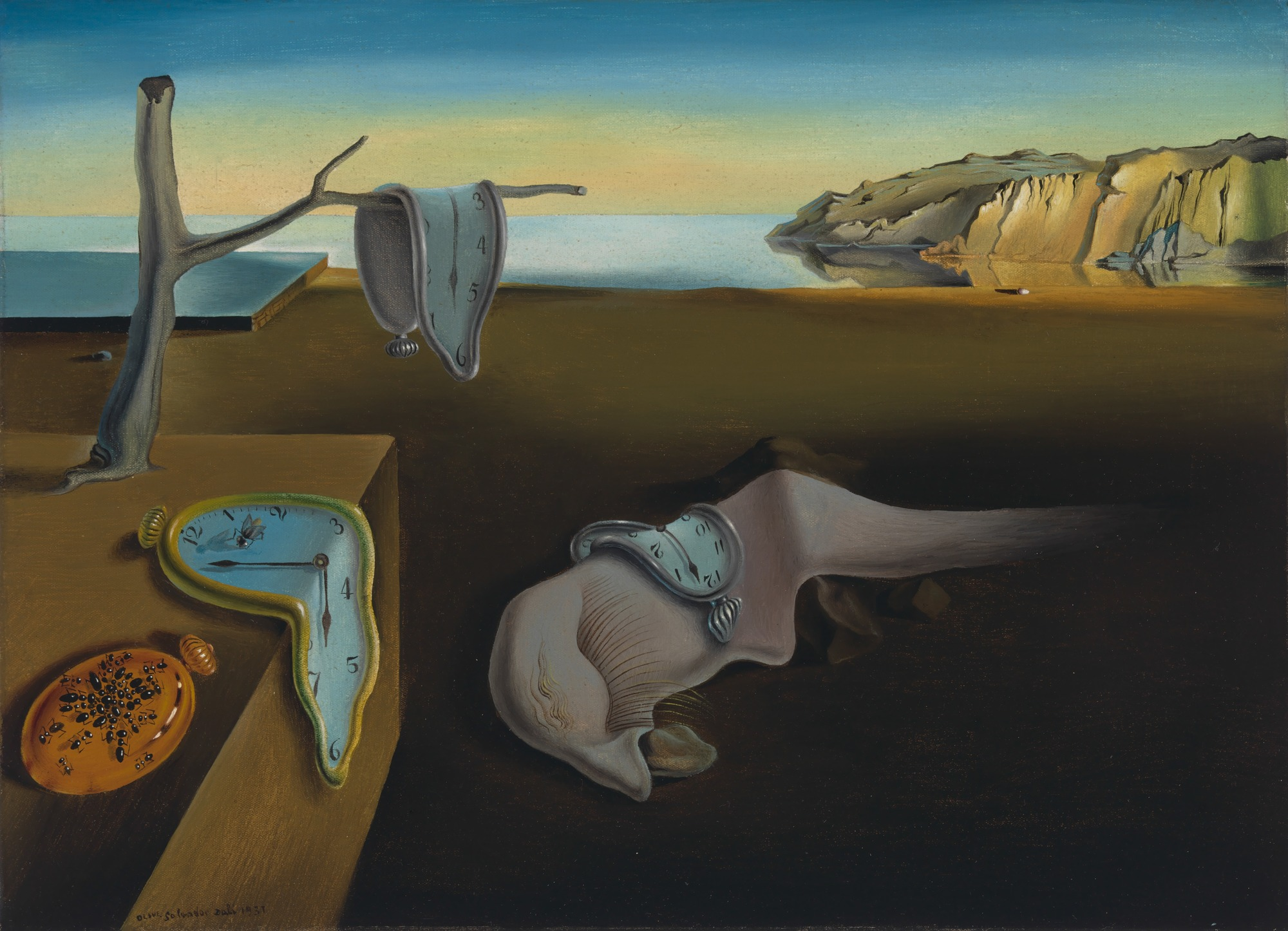## System clock

• 'long System.currentTimeMillis()' is the number of milliseconds that have elapsed since midnight on January 1, 1970 on the Greenwich Meridian minus the number of leap seconds, in terms of system clock.

• Granularity can be greater than one millisecond.

• "Freezes" when inserting a leap second.

• Can "jump" in both directions when synchronizing via NTP!

• 'long System.nanoTime()' — monotonous clock.

• Nanosecond accuracy.

• There is no reference to the "calendar" time.

• Over long periods, inaccuracy of the clock may become noticeable.

## When to use what?

• 'long System.nanoTime()' — when measuring time periods between events occurred on a single machine.

• But to measure the performance of Java programs, you should use JMH.

• 'long System.currentTimeMillis()' — to generate time stamps (minding the constraints).

## DateTime API

• Obsolete, inconvenient, mutable, non-currently safe classes (do not use them):

• Java 1.0 'Date' (it’s still with us because of JDBC),

• Java 1.1 `Calendar`.

• 'DateFormat' works with them.

• Modern (Java 8+), immutable classes, learned from mistakes:

• `LocalDate`, `LocalTime`, `Instant`, `Duration`, `Period`

• `ZoneId`, `ZonedDateTime`.

• 'DateTimeFormatter' works with them.

## `Instant`

• Point on time line

• UNIX time

``````//Current instant
Instant.now();
//explicitly specified Instant
Instant.ofEpochSecond(3);
//What year is it today?
int year = Instant.now().get(ChronoField.YEAR);``````

## `LocalDate`, `LocalTime` and `LocalDateTime`

• Date and time in the "human" sense.

• Without information about time zone → without reference to 'Instant'.

• Often binding to an Instant is not needed: "weekly call at 9:00" bound to Instant will "creep" due to daylight saving time.

``````LocalDate date = LocalDate.of(2014, 3, 18); // March 18, 2014

int year      = date.getYear(); //2014
Month month   = date.getMonth();  //MARCH
int day       = date.getDayOfMonth(); //18
DayOfWeek dow = date.getDayOfWeek(); //TUESDAY``````

## `LocalTime` and `LocalDateTime`

``````LocalTime time = LocalTime.of(13, 45, 20); //13:45:20

int hour   = time.getHour();
int minute = time.getMinute();
int second = time.getSecond();

LocalDateTime dt1 = LocalDateTime.of(2014, Month.MARCH, 18, 13, 45, 20);
LocalDateTime dt1 = LocalDateTime.of(date, time);
LocalDateTime dt2 = date.atTime(time);
LocalDateTime dt3 = time.atDate(date);

dt1.toLocalDate(); //2014-03-18
dt1.toLocalTime(); //13:45:20``````

## `Duration` and `Period`

• 'Duration': The amount of time between two points on a time line with nanosecond precision.

• 'Period': the time difference between `LocalDate`s in days (e.g. 'how many days is the contract valid?'

• Both implement the `TemporalAmount` interface.

``````//LocalTime, LocalDateTime, and Instant are suitable as arguments
Duration d = Duration.between(time1, time2);

//Only LocalDate is suitable as an argument
Period tenDays = Period.between(
LocalDate.of(2014, 3, 8),
LocalDate.of(2014, 3, 18));``````

## Constructing new objects from old ones

``````Instant i = Instant.now();
Duration d = Duration.ofSeconds(3);

//For example:
i.plus(dd.multipliedBy(10))
.minus(dd.minus(Duration.ofMillis(1)));

//the same can be done with LocalDateTime``````

## Format and parse date and time

``````DateTimeFormatter formatter1 =
DateTimeFormatter.ISO_LOCAL_DATE; // YYY-MM-DD
DateTimeFormatter formatter2 =
DateTimeFormatter.ofPattern("dd/MM/yyyy");
DateTimeFormatter formatter3 = new DateTimeFormatterBuilder()
.appendText(ChronoField.DAY_OF_MONTH)
.appendLiteral(". ")
.appendText(ChronoField.MONTH_OF_YEAR)
.appendLiteral(" ")
.appendText(ChronoField.YEAR)
.parseCaseInsensitive()
.toFormatter(Locale.ITALIAN);``````

## To string and from string

``````LocalDate date = LocalDate.of(2014, 3, 18);
String s = date.format(
DateTimeFormatter.ISO_LOCAL_DATE); // 2014-03-18
LocalDate date2 = LocalDate.parse(
s, DateTimeFormatter.ISO_LOCAL_DATE);``````

## Time zones

``````ZoneId moscowZone = ZoneId.of("Europe/Moscow");
ZoneId romeZone = ZoneId.of("Europe/Rome");
LocalDateTime dateTime = LocalDateTime.now();

ZonedDateTime moscowTime = dateTime.atZone(moscowZone);
System.out.println(moscowTime);
//2019-09-30T18:15:52.670+03:00[Europe/Moscow]

ZonedDateTime romeTime =
moscowTime.withZoneSameInstant(romeZone);
System.out.println(romeTime);
//2019-09-30T17:15:52.670+02:00[Europe/Rome]``````